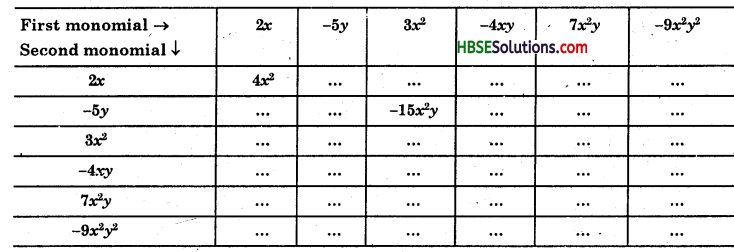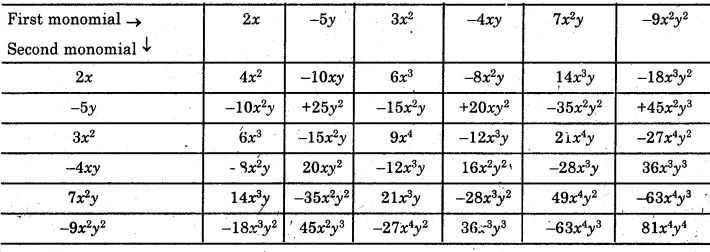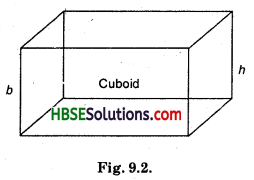# HBSE 8th Class Maths Solutions Chapter 9 Algebraic Expressions and Identities Ex 9.2

Haryana State Board HBSE 8th Class Maths Solutions Chapter 9 Algebraic Expressions and Identities Ex 9.2 Textbook Exercise Questions and Answers.

## Haryana Board 8th Class Maths Solutions Chapter 9 Algebraic Expressions and Identities Exercise 9.2

Question 1.
Find the product of the following pairs of monomials :
(i) 4, 7p
(ii) -4p, 7p
(iii) -4p, 7pq
(iv) 4p3, -3p
(v) 4p, 0.
Solution:
(i) 4 × 7p = (4 × 7) × p
= 28p.

(ii) -4p × 7p = (-4 × 7) × (p × p)
= -28p2.

(iii) -4p × 7pq = (-4 × 7) × (p × pq).
= -28p2q.

(iv) 4p3, -3p = [(4) × (-3)] × (p3 × p)
= -12 p4.

(v) 4p × 0 = (4 × 0) × p = 0 × p = 0.

Question 2.
Find the areas of rectangles with the following pairs of monomials as their lengths and breadths respectively.
(p, q); (10m, 5n); (20x2, 5y2); (4x, 3x2); (3mn, 4np)
Solution:
Area of rectangle = l × b sq. unit
(i) If l = p, b = q
Area of rectangle = p × q= pq sq.\unit.

(ii) If l = 10m, b = 5n
Area of rectangle = 10m × bn
= 50mn sq. unit.(iii) If l = 20x2, b =-5y2
Area of rectangle = 20x2 × 5y2
= 100x2y2 sq. unit

(iv) If l = 4x, b = 3x2
Area of rectangle = 4x × 3x2 = 12x3sq. unit

(v) If l = 3mn, b = 4np
Area of rectangle = 3mn × 4np
= 12mn2p sq. unit.

Question 3.
Complete the table of products.Solution:
Complete the table of products as shown in Table :Question 4.
Obtain the volume of rectangular boxes with the following length, breadth and height respectively:
(i) 5a, 3a2, 7a4,
(ii) 2p, 4p, 8r,
(iii) xy, 2x2y, 2xy2,
(iv) a, 2b, 3c.
Solution:
Volume of cuboid = l × b × h(i) l = 5a, b = 3a2, h = 7a4
Volume of rectangular box
= l × b × h cubic unit
= 5a × 3a2 × 7a4
= (5 × 3 × 7) × (a × a2 × a4)
= 105a7 cubic unit.

(ii) l = 2p, b = 4q, h = 8r
v = l × b × h, = 2p × 4q × 8r
= (2 × 4 × 8) × (p × q × r)
= 64pqr.(iii) l = xy, b = 2x2y, h = 2xy2
v = xy × 2x2y × 2xy2
= (1 × 2 × 2) × (xy × x2y × xy2)
= 4x4y4.

(iv) l = a, b = 2b, h = 3c
v = l × b × h = a × 2b × 3c
= (1 × 2 × 3) (a × b × c) = 6abc.

Question 5.
Obtain the product of
(i) xy, yz, zx
(ii) a, -a2, a3
(iii) 2, 4y, 8y2, 16y3
(iv) a, 2b, 3c, 6abc
(v) m, -mn, mnp.
Solution:
(i) xy × yz × zx = x2y2z2.
(ii) a × (-a2) × (a3) = -a6.

(iii) 2 × 4y × 8y2 × 16y3
= (2 × 4 × 8 × 16) × (y × y2 × y3)
= 1024 y6.(iv) a × 2b × 3c × 6abc
= (2 × 3 × 6) x (a x b × c × abc)
= 36a2b2c2.

(v) mx (-mn) × mnp = -m3n2p.